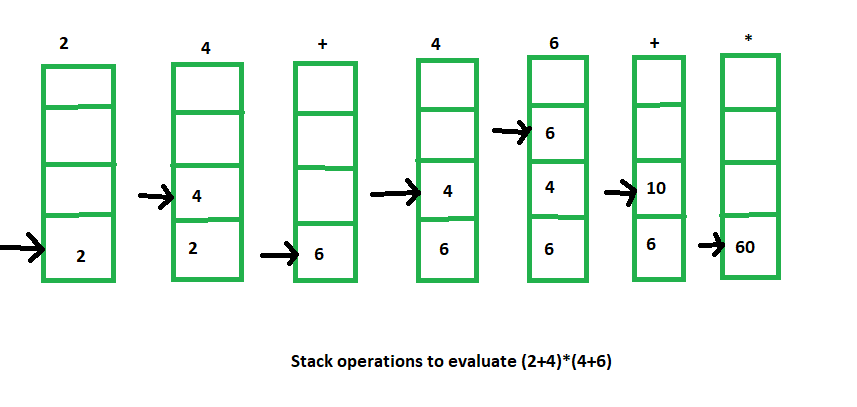GeeksforGeeks App
Open AppBrowser
Continue

# Arithmetic Expression Evaluation

The stack organization is very effective in evaluating arithmetic expressions. Expressions are usually represented in what is known as Infix notation, in which each operator is written between two operands (i.e., A + B). With this notation, we must distinguish between ( A + B )*C and A + ( B * C ) by using either parentheses or some operator-precedence convention. Thus, the order of operators and operands in an arithmetic expression does not uniquely determine the order in which the operations are to be performed.

1. Polish notation (prefix notation) –
It refers to the notation in which the operator is placed before its two operands. Here no parentheses are required, i.e.,

`+AB `

2. Reverse Polish notation(postfix notation) –
It refers to the analogous notation in which the operator is placed after its two operands. Again, no parentheses is required in Reverse Polish notation, i.e.,

`AB+ `

Stack-organized computers are better suited for post-fix notation than the traditional infix notation. Thus, the infix notation must be converted to the postfix notation. The conversion from infix notation to postfix notation must take into consideration the operational hierarchy.

There are 3 levels of precedence for 5 binary operators as given below:

```Highest: Exponentiation (^)
Next highest: Multiplication (*) and division (/)
Lowest: Addition (+) and Subtraction (-) ```

For example –

```Infix notation: (A-B)*[C/(D+E)+F]
Post-fix notation: AB- CDE +/F +* ```

Here, we first perform the arithmetic inside the parentheses (A-B) and (D+E). The division of C/(D+E) must be done prior to the addition with F. After that multiply the two terms inside the parentheses and bracket.

Now we need to calculate the value of these arithmetic operations by using a stack.

The procedure for getting the result is:

1. Convert the expression in Reverse Polish notation( post-fix notation).

2. Push the operands into the stack in the order they appear.

3. When any operator encounters then pop two topmost operands for executing the operation.

4. After execution push the result obtained into the stack.

5. After the complete execution of expression, the final result remains on the top of the stack.

For example –

```Infix notation: (2+4) * (4+6)
Post-fix notation: 2 4 + 4 6 + *
Result: 60 ```

The stack operations for this expression evaluation is shown below:My Personal Notes arrow_drop_up